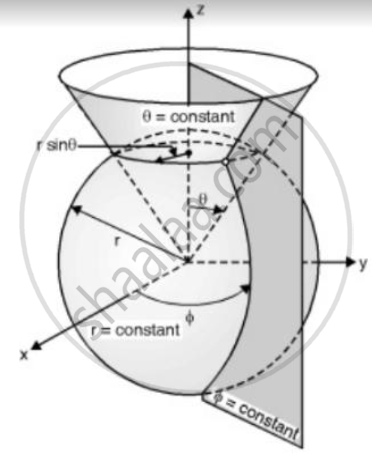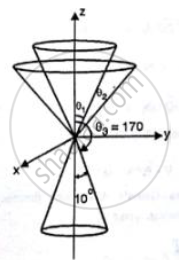# Explain Spherical Co-ordinate System. State the Transformation Relation Between Cartesian and Spherical Co-ordinates. - Applied Physics 2

Explain spherical co-ordinate system. State the transformation relation between Cartesian and spherical co-ordinates.

#### Solution

For spherical coordinates system also x, y, z axes are used for reference. Imagine a sphere of radius r with centre at origins. Any point on the sphere is ta the same distance r from origin, therefore the spherical surface is defined
as r= constant surface.
Now consider a line from origin making angle θ with z-axis. Rotate this line about z-axis fixing the end at the origin. This forms a cone with angle θ, this conical surface is defined as a θ = constant surface. When a sphere with centre at origin intersects with the vertical cone with vertex at origin, the intersection is a horizontal circle with radius equal to r sin θ. We want to locate a point in spherical coordinate system. Imagine a ∅ = constant plane similar to in cylindrical system. A horizontal circle with centre onz-axis, ∅=constant plane. The intersection is the point.
Because r=constant, θ=constant and ∅=constant surface intersects at a point, the point is defined as (r, ∅, θ). In spherical system varriations of angle θ is from 0 to 1800 and variation of ∅ is from 0 to 3600Conversion between cartesian and spherical coordinates:

The unit vector in spherical system are ar, aθ, a. These unit vectors are perpendicular to r= constant, θ=constant, ∅=constant surface respectively and in the increasing directions of the r, θ, ∅ respectivley.When point is present in cartesian system, it must have corresponding cartesian coordinates. Here we are going to find relations between spherical coordinates. To find x and y corrsponding to point p, project point p in xy plane. The  projection is OQ = rsinθ
Projection of OQ on x-axis is
OR=OQcos∅=rsinθcos∅
∴x= rsinθcos∅
Projection of OQ on y-axis is
OS= OQsin∅= rsinθsin∅
y= rsinθsin∅
projection of OP on z-axisgives z-coordinats of p
projection of OP on z-axis = OT= OPcosθ
z=rcosθ

Concept: Cylindrical and Spherical Coordinate System
Is there an error in this question or solution?